### Timed Worksheets - By Topics

Each specially designed timed worksheet for each topics (addition, subtraction, multiplication, division, fraction, decimal, integer, and equation), allows students to start problem solving at their own ability level. Students will gradually move step-by-step until they master every math concept.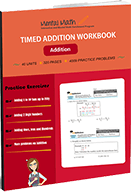Adding up to 5 digit Numbers and 3 Numbers

See all units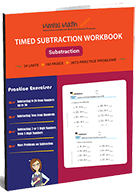### Timed Subtraction Worksheets

Subtracting 4 to 1-digit Numbers form 4 digit Numbers

See all units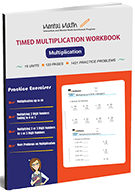### Timed Multiplication Worksheets

Multiplication Table of 2 through 10 and Multiplication by Thousands

See all units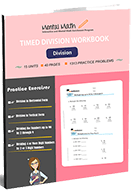### Timed Division Worksheets

Dividing up to 4 or more digit Numbers by 2 or 3-digit Numbers

See all units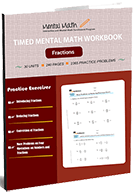### Timed Fractions Worksheets

Fraction Concepts and Four Operations on Fractions

See all units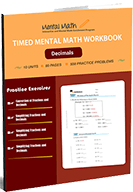### Timed Decimals Worksheets

Decimal Concept and Four Operations on Decimals

See all units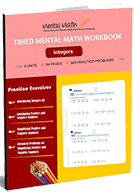### Timed Integers Worksheets

Four Operations on integers and their Simplification

See all units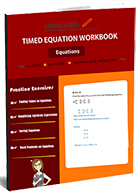### Timed Equation Worksheets

Evaluate Algebraic Expressions and Solve Equations

See all units